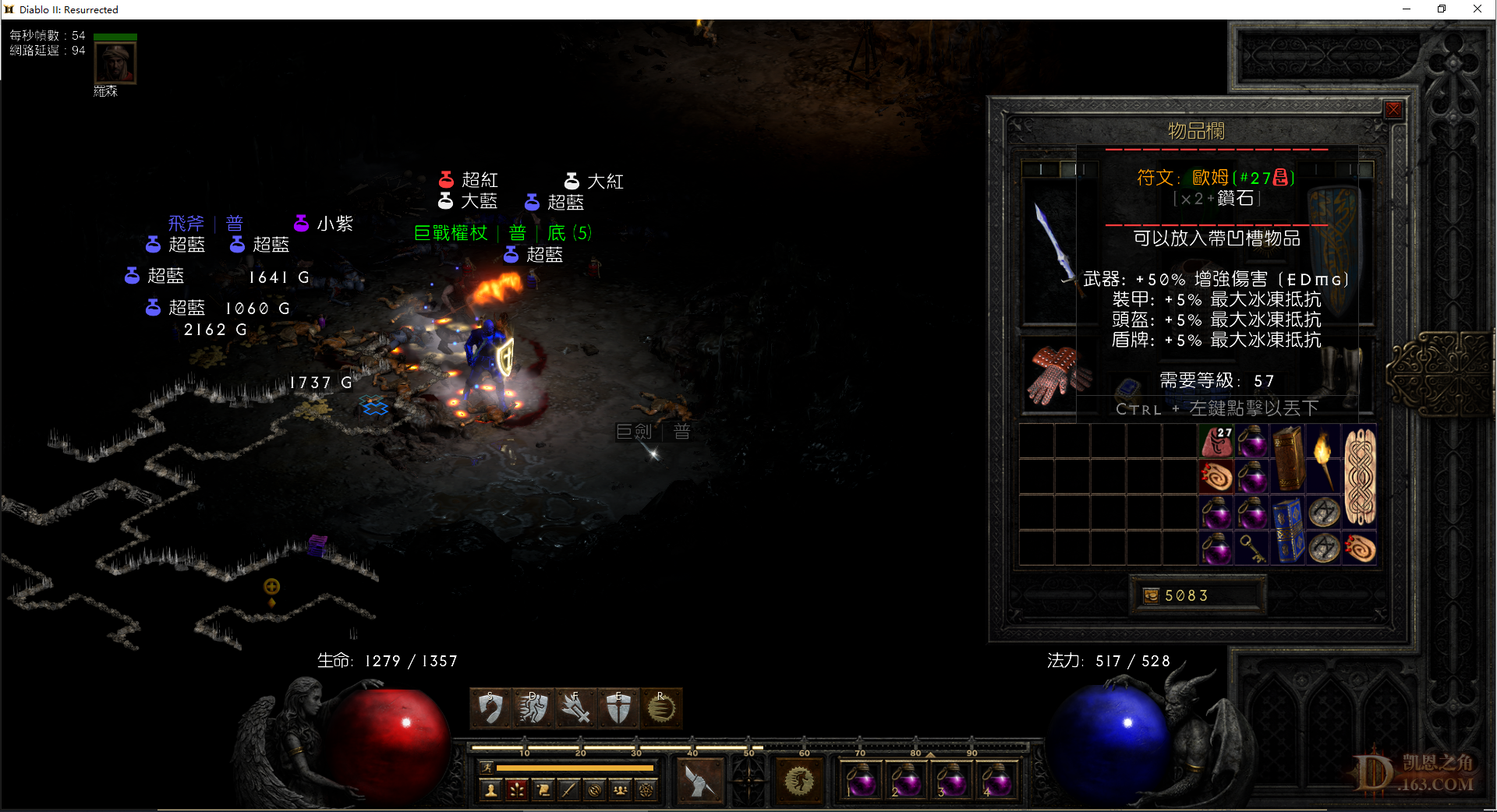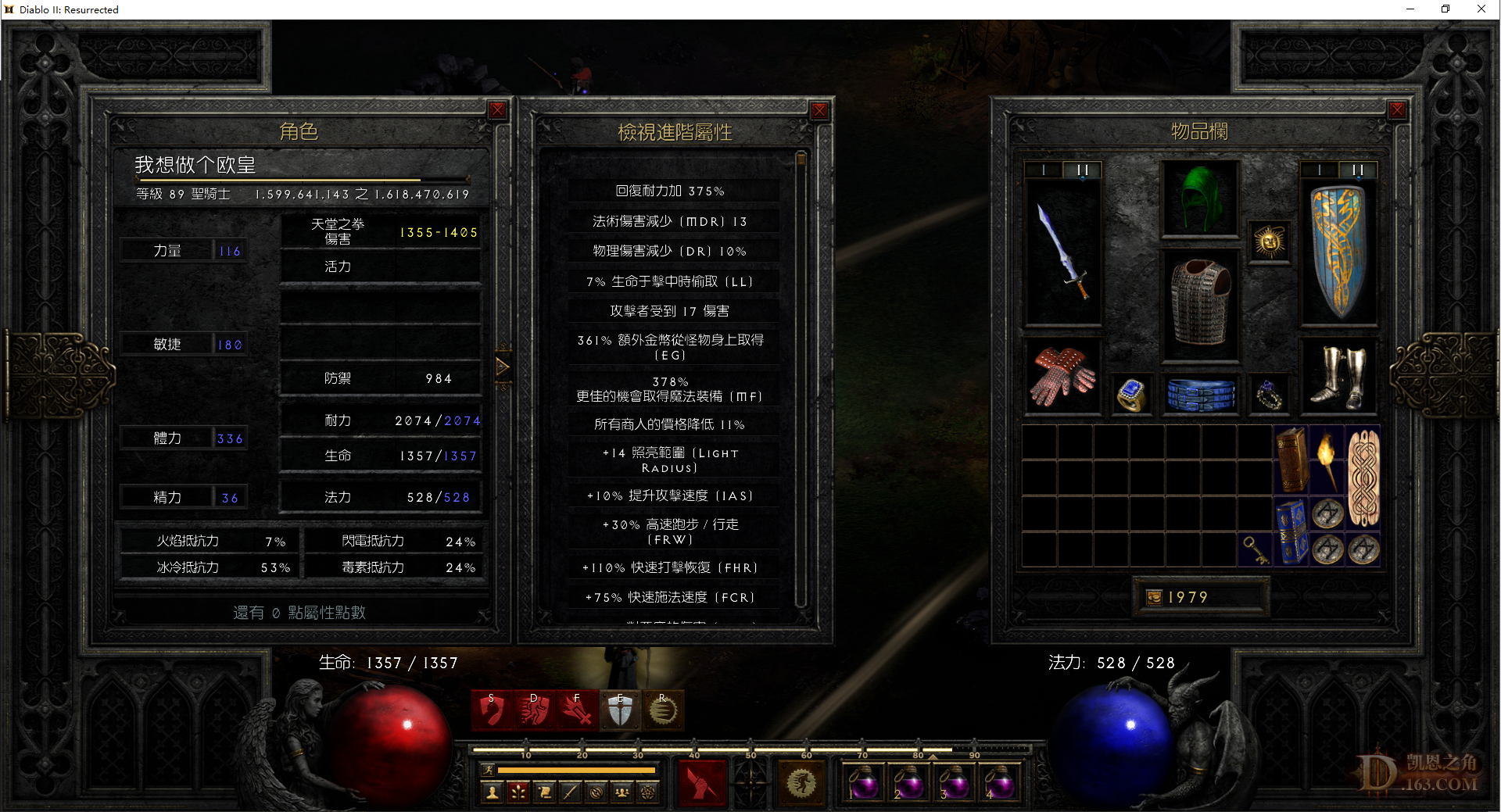//没登陆

 341~350：空。 感觉没达到凯恩平均水平。。。。。。。

33=0，32=0，31=0，30=0，29=0，28=0，27=3，26=0，25=0，24=2，格里风=0，次元=0，破隐=1，年纪=0，乔丹=2。
 351~360：空。 黎明前的黑暗吗？33=0，32=0，31=0，30=0，29=0，28=0，27=3，26=0，25=0，24=2，格里风=0，次元=0，破隐=1，年纪=0，乔丹=2。
 70级就厉害了，多少mf啊，出了好多

 Hansonwu 发表于 2022-5-12 16:56 70级就厉害了，多少mf啊，出了好多 天堂之拳伤害很高，70级够了，绰绰有余。刚开始打的时候，200多MF，现在装备上来了，MF373。但感觉没什么区别。

33=0，32=0，31=0，30=0，29=0，28=0，27=3，26=0，25=0，24=2，格里风=0，次元=0，破隐=1，年纪=0，乔丹=2。
 本帖最后由 黄黄黄 于 2022-5-12 20:53 编辑 361~370：27#（出货了，出货了，终于出货了。。。。），3孔41抗神圣轻圆盾，小妖精脚趾。 终于又出高级符文了，看来非洲人还是要靠多刷。。。。。33=0，32=0，31=0，30=0，29=0，28=0，27=3，26=0，25=0，24=2，格里风=0，次元=0，破隐=1，年纪=0，乔丹=2。
 371~380：死灵诅咒板。

33=0，32=0，31=0，30=0，29=0，28=0，27=3，26=0，25=0，24=2，格里风=0，次元=0，破隐=1，年纪=0，乔丹=2。
 381~390：20敏乌鸦，玛那得的治疗。

33=0，32=0，31=0，30=0，29=0，28=0，27=3，26=0，25=0，24=2，格里风=0，次元=0，破隐=1，年纪=0，乔丹=2。
 本帖最后由 黄黄黄 于 2022-5-13 14:18 编辑 391~400：塔拉夏头，黑色黑帝斯。 400把了，不容易啊。5月6日开始到现在，刚好一个星期。70级开始，到现在差一格半就90级了。400把出了两个27#，1个22#，1个23#，两个安头，一个军帽，还有个5fhr5r小符。感觉不算黑，但跟那些10把就出贝的大佬差远了。继续刷吧，目标无限格里风，然后起飞我的法师~~~33=0，32=0，31=0，30=0，29=0，28=0，27=3，26=0，25=0，24=2，格里风=0，次元=0，破隐=1，年纪=0，乔丹=2。
 401~410：空。

33=0，32=0，31=0，30=0，29=0，28=0，27=3，26=0，25=0，24=2，格里风=0，次元=0，破隐=1，年纪=0，乔丹=2。
 411~420：巫师之刺，拿各。

33=0，32=0，31=0，30=0，29=0，28=0，27=3，26=0，25=0，24=2，格里风=0，次元=0，破隐=1，年纪=0，乔丹=2。
 421~430：空。

33=0，32=0，31=0，30=0，29=0，28=0，27=3，26=0，25=0，24=2，格里风=0，次元=0，破隐=1，年纪=0，乔丹=2。
 超市今天最大25

 35基德和20敏乌鸦出吗？27号换无形丧钟可以吗？